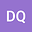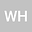••In this paper, the cubic B-spline element method is proposed for a class of fourth order nonlinear parabolic problem with variable coefficient. We prove the boundness of the approximate solutions of the semi-discrete and fully discrete finite element schemes. The boundness is the basis of error analysis of nonlinear parabolic problem, especially in the case of fourth order term with variable coefficient. The error estimates are discussed by constructing the energy functional in $L^2$ norm and $H^2$ norm. Numerical results confirm our results of theoretical analysis.